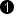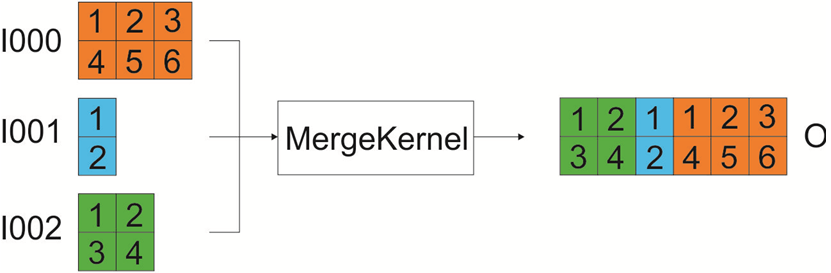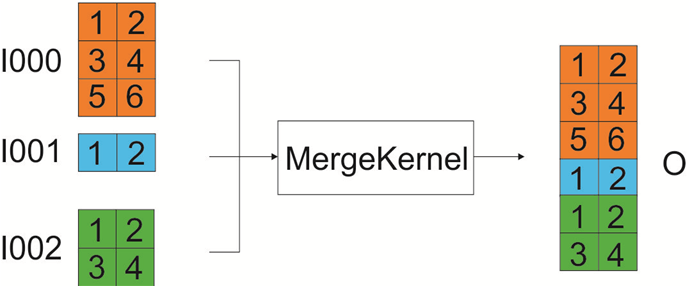## Operator MergeKernel

The operator MergeKernel merges an arbitrary number N of input links I .. I (max.) into a single output link O.

The operator allows kernels at its inputs. One kernel dimension (row or column) needs to have the same size (number of pixels) at all inputs. For the other dimension (rows or columns) an individual size can be defined for each input. Thus, either the number of rows or the number of columns has to be the same for all kernels at the inputs.

For detailed information, see parameter description.

### I/O Properties

Property Value
Operator Type O
Input Links I, data input
I[n], n > 0, data input
Output Link O, data output

### Supported Link Format

Link Parameter Input Link I Input Link I[n], n > 0 Output Link O
Bit Width [1, 64]as I as I
Arithmetic {unsigned, signed} as I as I
Parallelism any as I as I
Kernel Columns any horizontal: any
vertical: as I
horizontal: sum of all kernel columns
vertical: as I
Kernel Rows any horizontal: as I
vertical: any
horizontal: as I
vertical: sum of all kernel rows
Img Protocol {VALT_IMAGE2D, VALT_LINE1D, VALT_PIXEL0D} as I as I
Color Format any as I as I
Color Flavor any as I as I
Max. Img Width any as I as I
Max. Img Height any as I as I
 The range of the input bit width is [1, 64]. For signed inputs, the range is [2, 64]. For unsigned color inputs, the range is [3, 63] and for signed color, the range is [6, 63].

### Parameters

Alignment
Type static write parameter
Default horizontal
Range [horizontal, vertical]

Parameter Alignment defines the ordering of the output vector. The vector is a concation of all inputs in either horizontal or vertical direction.

Via parameter Alignment, you define if the number of rows or the number of columns has the same value for all kernels at the inputs.

Horizontal: If you select "horizontal" (default) the number of rows needs to be the same for the incoming kernels at all inputs. The number of columns you can define individually for each incoming kernel. In the output, the number of rows is the same as in the inputs. The number of columns will be the sum of the columns of all inputs.Vertical: If you select "vertical" the number of rows can be defined individually for each incoming kernel. The number of columns needs to be the same for the incoming kernels at all inputs. In the output, the number of columns is the same as in the inputs. The number of rows will be the sum of the rows of all inputs.### Examples of Use

The use of operator MergeKernel is shown in the following examples: